Study S4 Chemistry Energy Changes - Geniebook# Energy Changes

• Describe the term exothermic as a process or chemical reaction which transfers energy, often in the form of heat, to the surroundings.
• Describe the term endothermic as a process or chemical reaction which takes in energy, often in the form of heat, from the surroundings.

## Energy Changes

All chemical reactions involve energy changes, when energy is either absorbed from the surroundings or released to the surroundings.

Some physical changes also involve energy changes.

When energy change occurs, a temperature change in the surroundings can be observed.

### Experiment To Investigate Energy Change

######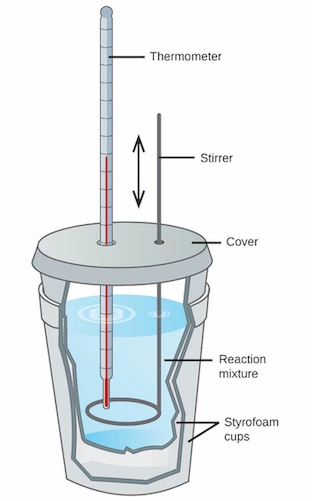Image Credit: OpenStax Resource

The above set−up can be used to investigate energy changes.

Water is added to a styrofoam cup and its temperature is first measured using a thermometer. Then, a solid substance is placed into the water in the cup and the mixture is stirred. After the solid has completely dissolved, the temperature of the water is measured again.

A temperature change will indicate that heat energy is either absorbed from the surroundings or released to the surroundings.

#### Why do we use a styrofoam cup with a cover?

As styrofoam is a poor conductor of heat, it helps to minimise heat loss to the surroundings. Hence, we can obtain a more accurate measurement of the temperature change.

#### Why do we use a stirrer in this experiment?

Stirring will ensure the reactants are mixed properly. It also ensures that the heat is evenly distributed throughout the mixture.

Let us now look at the enthalpy change in terms of exothermic and endothermic reactions associated with energy transfer to/from the surroundings.

## Exothermic Changes

An exothermic change is a change in which heat is given out to the surroundings.

Heat is released from the reactants and transferred to the surroundings. The temperature in the surroundings rises.

The surroundings can refer to the container, surrounding air and the reaction mixture.

######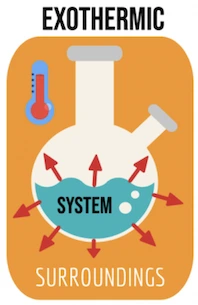Image Credit: Let’s Talk Science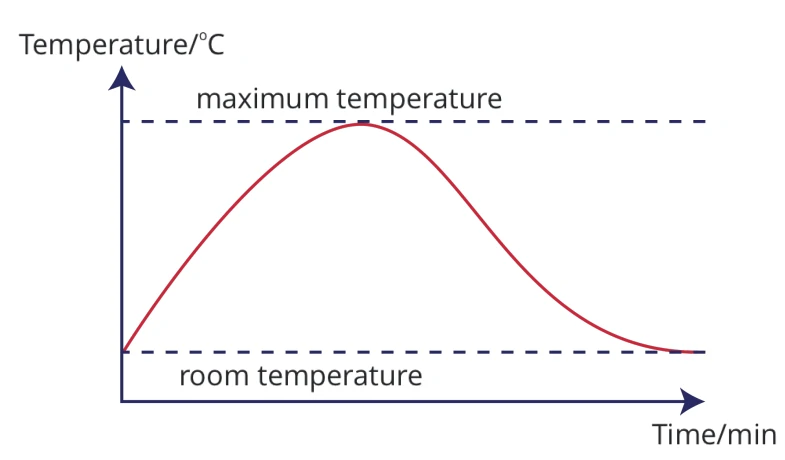Take the example of dilute hydrochloric acid reacting with sodium hydroxide solution. When an exothermic change occurs, the temperature of the reaction mixture initially increases until the maximum temperature is reached (i.e. the reaction has gone to completion). Once the reaction is completed, the temperature of the reaction mixture will start to fall until it reaches room temperature.

### Chemical Reactions

• #### Combustion Of Fuels

Example: Burning of methane

$$\mathrm{CH_4 + 2O_2 \quad\xrightarrow[]{\qquad\qquad}\quad CO_2 + 2H_2O}$$

• #### Respiration

Respiration is a type of combustion reaction in living cells, in which simple sugars are burnt in the presence of oxygen to release energy.

$$\mathrm{C_6H_{12}O_6 + 6O_2 \quad\xrightarrow[]{\qquad\qquad}\quad 6CO_2 + 6H_2O}$$

• #### Neutralisation

Example: Reaction between dilute hydrochloric acid and sodium hydroxide solution.

$$\mathrm{HCl + NaOH \quad\xrightarrow[]{\qquad\qquad}\quad NaCl + H_2O}$$

• #### Corrosion Of Metals

Example: Rusting of iron, in the presence of oxygen and water

### Physical Processes

• #### Condensation

Example: When water vapour condenses into liquid water

$$\mathrm{H_2O\;(g) \quad\xrightarrow[]{\qquad\qquad}\quad H_2O\;(l)}$$

• #### Freezing

Example: When liquid water turns into solid ice

$$\mathrm{H_2O\;(l) \quad\xrightarrow[]{\qquad\qquad}\quad H_2O\;(s)}$$

## Endothermic Changes

An endothermic change is a change in which heat is absorbed from the surroundings.

Heat energy is absorbed and transferred from the surroundings to the reactants. The temperature of the surrounding falls.

######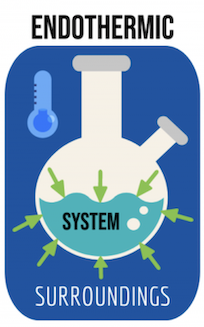Image Credit: Let’s Talk Science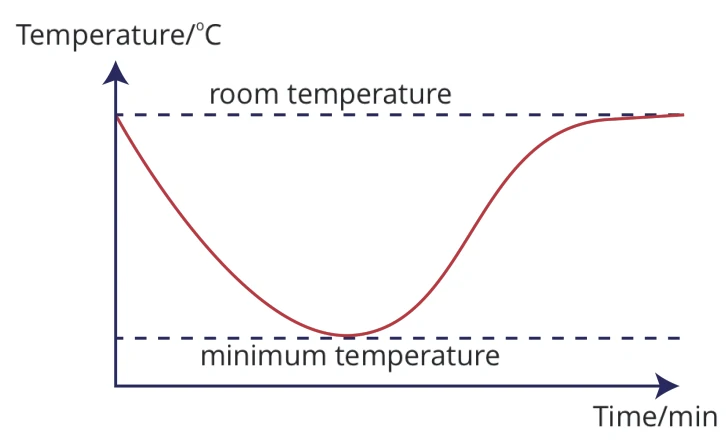Take the example of solid ammonium nitrate dissolving in water. When the endothermic change occurs, the temperature of the reaction mixture falls until the minimum temperature is reached (i.e. the reaction has gone to completion). When the reaction is completed, the temperature of the reaction mixture will rise again until it reaches room temperature.

### Chemical Reactions

• #### Photosynthesis

$$\mathrm{6CO_2 + 6H_2O \quad\xrightarrow[]{\qquad\qquad}\quad C_6H_{12}O_6 + 6O_2}$$

• #### Thermal Decomposition

Example: The breaking down of calcium carbonate to form calcium oxide

$$\mathrm{CaCO3 \quad\xrightarrow[]{\qquad\qquad}\quad CaO + CO_2}$$

• #### Action of light on silver bromide in photographic film

$$\mathrm{2AgBr \quad\xrightarrow[]{\qquad\qquad}\quad 2Ag + Br_2}$$

• #### Evaporation/ boiling

Example: liquid water turning into water vapour/steam

$$\mathrm{H_2O\;(l) \quad\xrightarrow[]{\qquad\qquad}\quad H_2O\;(g)}$$

• #### Melting

Example: solid ice becoming liquid water

$$\mathrm{H_2O\;(s) \quad\xrightarrow[]{\qquad\qquad}\quad H_2O (l)}$$

• #### Dissolving of some ionic compounds in water

Example: dissolving ammonium nitrate in water

$$\mathrm{NH_4NO_3\;(s) \quad\xrightarrow[]{\qquad\qquad}\quad NH_{4}^{ +}\;(aq) + NO_{3}^{−}\;(aq)}$$

######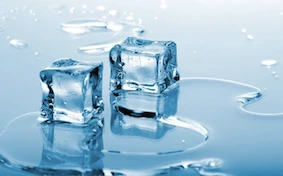Image Credit: FinTech Sandbox

Answer the following questions based on the concepts that we have covered in this article. If you are unclear, you may want to revisit the relevant section to revise the concepts.

Question 1:

Which of the following statements is true for an exothermic reaction?

1. Energy is absorbed from the surroundings and temperature decreases.
2. Energy is absorbed from the surroundings and temperature increases.
3. Energy is released to the surroundings and temperature decreases.
4. Energy is released to the surroundings and temperature increases.

Solution:

(D) Energy is released to the surroundings and temperature increases.

Explanation:

During an exothermic reaction, energy in the form of heat is released to the surroundings, causing the surrounding temperature to increase.

Question 2:

Commercially available ‘cold packs’ and ‘heat packs’ use reactions to cause a change in temperature. One type of pack consists of two bags; an inner bag containing water, and an outer bag containing ammonium nitrate. When the inner bag of water is broken by squeezing the package, water that is released dissolves the ammonium nitrate. When this occurs, the temperature of the solution decreases. Based on the information provided, which of the following should ammonium nitrate be used?

1. cold pack, because it undergoes an endothermic dissolution process
2. cold pack, because it undergoes an exothermic dissolution process
3. heat pack, because it undergoes an endothermic dissolution process.
4. heat pack, because it undergoes an exothermic dissolution process

Solution:

(A) cold pack, because it undergoes an endothermic dissolution process

Explanation:

An endothermic reaction is a chemical reaction in which heat energy is absorbed from the surroundings. Thus the temperature of the surroundings will decrease. Since the temperature of the solution in this pack decreases, the dissolution of ammonium nitrate in water is endothermic.

Question 3:

Which of the following processes is endothermic?

2. burning of coal in the air
3. dissolving of ammonium nitrate in water
4. reaction between an acid and an alkali

Solution:

(C) dissolving of ammonium nitrate in water

Explanation:

An endothermic process is one in which heat energy is absorbed from the surroundings.
Ammonium nitrate is an ionic compound which absorbs heat energy from the surroundings when dissolved in water. Hence, it is an endothermic process.
On the other hand, combustion (burning of coal in air), neutralisation (between an acid and an alkali) as well as the reaction of a reactive metal with water are exothermic processes, in which heat energy is released or given out to the surroundings.

Question 4:

Which of the following processes is different from the others in terms of energy changes?

1. combustion
2. neutralisation
3. photosynthesis
4. respiration

Solution:

(C) photosynthesis

Explanation:

Combustion, neutralisation and respiration are examples of exothermic reactions, while photosynthesis is an example of an endothermic reaction.

Question 5:

Dissolving ammonium nitrate in water is endothermic. Which graph shows how the temperature changes as ammonium nitrate is added to water and then the solution is left to stand?

 (1)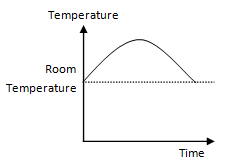(2)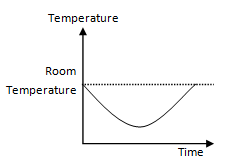(3)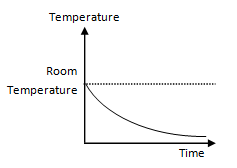(4)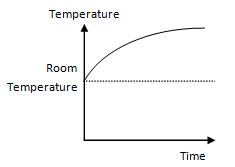Solution:

(2)

Explanation:

Since the reaction is endothermic, heat is absorbed from the surroundings. The temperature of the surroundings will drop until all the ammonium nitrate has completely reacted with water. The temperature will then increase again until it returns back to room temperature when the solution is left to stand.

Question 6:

Which of the following reactions is endothermic?

1.   $$\mathrm{CO_2\;(g) \quad\xrightarrow[]{\qquad\qquad}\quad CO_2\;(s)}$$
2.   $$\mathrm{MgCO_3\;(s) \quad\xrightarrow[]{\qquad\qquad}\quad MgO\;(s) + CO_2\;(g)}$$
3.   $$\mathrm{C\;(s) + O_2\;(g) \quad\xrightarrow[]{\qquad\qquad}\quad CO_2\;(g)}$$
4.   $$\mathrm{H_2O\;(l) \quad\xrightarrow[]{\qquad\qquad}\quad H_2O\;(s)}$$

Solution:

(B)   $$\mathrm{MgCO_3\;(s) \quad\xrightarrow[]{\qquad\qquad}\quad MgO\;(s) + CO_2\;(g)}$$

Explanation:

Option 1: represents a deposition process in which carbon dioxide gas turns into dry ice (solid carbon dioxide). This process involves the release of heat energy to the surroundings, hence it is exothermic.

Option 2: represents the thermal decomposition of $$\mathrm{MgCO_3}$$, which requires heat energy in order to occur, hence it is endothermic.

Option 3: represents the combustion of carbon to form carbon dioxide, in which heat energy is released to the surroundings, hence it is exothermic.

Option 4: represents the freezing of liquid water into solid ice. This process also involves heat energy being released to the surroundings, hence it is exothermic.

## Conclusion

In this article, we learnt about characteristics of exothermic and endothermic changes, as well as some examples of these energy changes. The article will help the students of Upper Secondary Chemistry class to grasp and better understand these concepts.

Continue Learning
Metals Introduction to Organic Chemistry
Alcohols Speed of Reaction
Electrolysis Energy Changes
Ammonia Alkanes
Alkenes Carboxylic AcidsPrimaryPrimary 1Primary 2Primary 3Primary 4Primary 5Primary 6SecondarySecondary 1Secondary 2Secondary 3Secondary 4PhysicsChemistry
Carboxylic Acids
Metals
Introduction to Organic Chemistry
Alcohols
Speed Of Reaction
Electrolysis
Energy Changes
Ammonia
Alkanes
AlkenesMaths